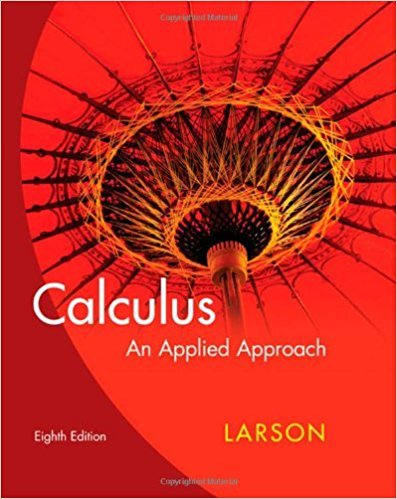×
×

# Solutions for Chapter 0.4: Factoring Polynomials## Full solutions for Calculus: An Applied Approach | 8th Edition

ISBN: 9780618958252Solutions for Chapter 0.4: Factoring Polynomials

Solutions for Chapter 0.4
4 5 0 247 Reviews
26
0
##### ISBN: 9780618958252

Chapter 0.4: Factoring Polynomials includes 78 full step-by-step solutions. This expansive textbook survival guide covers the following chapters and their solutions. Calculus: An Applied Approach was written by and is associated to the ISBN: 9780618958252. Since 78 problems in chapter 0.4: Factoring Polynomials have been answered, more than 21743 students have viewed full step-by-step solutions from this chapter. This textbook survival guide was created for the textbook: Calculus: An Applied Approach , edition: 8.

Key Calculus Terms and definitions covered in this textbook
• Ambiguous case

The case in which two sides and a nonincluded angle can determine two different triangles

• Angle

Union of two rays with a common endpoint (the vertex). The beginning ray (the initial side) can be rotated about its endpoint to obtain the final position (the terminal side)

• Base

See Exponential function, Logarithmic function, nth power of a.

• Cofunction identity

An identity that relates the sine, secant, or tangent to the cosine, cosecant, or cotangent, respectively

• Cubic

A degree 3 polynomial function

• Equilibrium point

A point where the supply curve and demand curve intersect. The corresponding price is the equilibrium price.

• Hyperbola

A set of points in a plane, the absolute value of the difference of whose distances from two fixed points (the foci) is a constant.

• Infinite sequence

A function whose domain is the set of all natural numbers.

• NDER ƒ(a)

See Numerical derivative of ƒ at x = a.

• Power-reducing identity

A trigonometric identity that reduces the power to which the trigonometric functions are raised.

• Range of a function

The set of all output values corresponding to elements in the domain.

• Real axis

See Complex plane.

• Real zeros

Zeros of a function that are real numbers.

• Reflection

Two points that are symmetric with respect to a lineor a point.

• Slope

Ratio change in y/change in x

• Supply curve

p = ƒ(x), where x represents production and p represents price

• Symmetric matrix

A matrix A = [aij] with the property aij = aji for all i and j

• Trichotomy property

For real numbers a and b, exactly one of the following is true: a < b, a = b , or a > b.

• Unit ratio

See Conversion factor.

• Ymax

The y-value of the top of the viewing window.

×